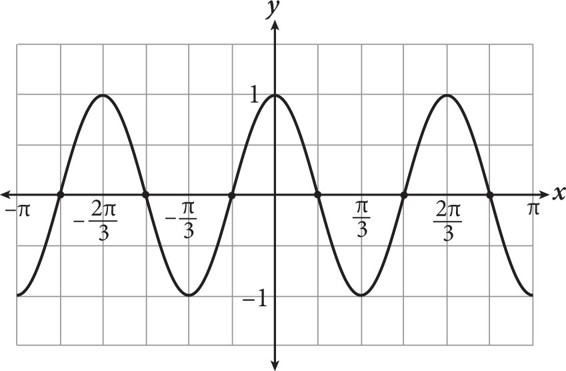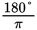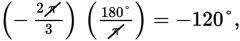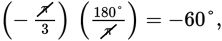# SAT Math Multiple Choice Question 299: Answer and Explanation

### Test Information

Question: 299

14.The graph of g(x) = cos(3x) is shown above. Which of the following lists represents the values of x for which g(x) = 0?

• A. –180°, –120°, –60°, 60°, 120°, 180°
• B. –165°, –105°, –45°, 45°, 105°, 165°
• C. –150°, –90°, –30°, 30°, 90°, 120°
• D. –120°, –80°, –40°, 40°, 80°, 120°

Strategic Advice: You don't need to know a lot of trig to answer this question. You will, however, need to know how to convert radians to degrees (multiply the radians by).
Getting to the Answer: Recall that g(x) = 0 means "crosses the x-axis," regardless of the type of function involved, which means you are looking for the x-intercepts. Study the graph carefully: The function crosses the x-axis six times, halfway between each of the labeled grid-lines. Rather than finding the points using the radians given in the graph, convert the radians to degrees and then determine the halfway points:and so on. Take a minute now to find the halfway points because chances are that you don't have to do all the conversions. Halfway between -180° and -120° is -150°. Stop-that's all you need to know. The leftmost x-intercept is at -150°, which means (C) must be correct. If you want to check another value just to be sure, halfway between -120° and -60° is -90°, which is the second value in (C), confirming that it is correct.